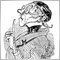# String conversion161

Hello!

is the following somehow possible?

```double num = 123.12;

string Arr[]={"num"};

StringToDouble(Arr);```

This results in 0.0 and not in 123.12

What obviously works but is not what I Need is:

```string test[]={"123.12"};

StringToDouble(test);```

This results in 123.12

Why I Need this is because it is not possible to initialize an Array with = { ..... } using variables such as A.Value where A is a class

So what I finally want to achieve is something like:

```string test[]={"A.Value"};

StringToDouble(test);```Moderator
9812

1. Du kannst hier deutsch sprechen!
2. Bei der Definition des Arrays kannst/darfst Du  keine Variablen verwenden!
3. Das sollte gehen (ungetestet!)
```#define num 123.12;
double Arr[]={num};
StringToDouble(DoubleToString(Arr));

// oder
double num = 123.12;
double Arr;
Arr = num;
StringToDouble(DoubleToString(Arr));

// oder
double num = 123.12;
double Arr[];
ArrayResize(Arr,1);
Arr = num;
StringToDouble(DoubleToString(Arr));
```161

Carl Schreiber:
1. Du kannst hier deutsch sprechen!
2. Bei der Definition des Arrays kannst/darfst Du  keine Variablen verwenden!
3. Das sollte gehen (ungetestet!)

Hallo Carl,

vielen Dank für die Ausführung. Variante 1 wäre perfekt aber das funktioniert leider auch nur mit Konstanten.

Wenn ich folgendes mache:

ClassTest A;

#define num A.Wert

meckert er, und sagt er braucht eine konstante und keine variable.Moderator
9812

Naja, so ist das halt: Du kannst nur machen was der Compiler Dir erlaubt.

Alles andere ist verlorene Zeit!161

Ja, ich belasse es jetzt auch dabei. Hab noch einiges versucht aber aktuell finde ich dazu keine Lösung.

Trotzdem vielen Dank für deine Unterstützung!

Liebe Grüße

Einloggen oder registrieren, um einen Kommentar zu schreiben